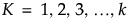The basic approach of outlier detection is to consider points distant from other points as outliers. One way of determining the distance of a point to other clusters of points is explore the distance to its nearest neighbors. For each value of K, the Multivariate k-Nearest Neighbor Outliers utility displays a plot of the Euclidean distance from each point to it’s Kth nearest neighbor. You specify the largest value of K, denoted as k. Plots are provided for, skipping values by the Fibonacci sequence to avoid displaying too many plots.
This approach is sensitive to the specified value of k. A small value of k can miss identifying points as outliers and a large value of k can falsely classify points as outliers:
 • Suppose that the specified k is small, so that you are only studying a few neighbors. If there is a cluster of more than k points that is far from the rest of the points, then the points within the cluster will have small distances to their nearest neighbors. You may be unable to detect the cluster of outliers.
 • Suppose that the specified k is large, so that you are studying a large number of neighbors. If there are clusters with fewer than k data points, then the points within these clusters may appear to be outliers. You may overlook the fact that the points form a cluster, interpreting the individual cluster members as outliers instead.
When you select Multivariate k-Nearest Neighbor Outliers from the list of commands, you are asked to specify the value of k to use as an upper bound for the furthest neighbor to be considered. Notice that the default value is set to 8.
The report shows plots for select values of K up to the value k. The value of K for each plot is displayed in its vertical axis label, which is of the form Distance to Neighbor K = <a>, where a is an integer denoting the ath closest neighbor. Each plot shows the distance from the point in the ith row to its ath nearest neighbor. The points that have large distances from their neighbors, across multiple values of K, are likely to be outliers.
Saves the distances from each row to its nth nearest neighbor as new columns in the data table.

Help created on 7/12/2018